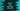# How to read excel data in python using xlrd## Python read excel data using xlrd:

To read a excel file in python, there are a couple of libraries available. xlrd is one of them and it is a popular library for handling excel file in python.

In this post, we will learn how to read data from a excel file using xlrd using a python script.

Note that xlrd works only with .xls files.

### Installation of xlrd:

xlrd can be installed using pip:

``pip install xlrd``

### xls file for the examples:

The below xls file I have created for the examples we are using here:

### Example 1: Reading number of rows and columns:

The file I created in above step is sample.xls and I put it in the same folder. The below code will print the total number of rows and total number of columns:

``````import xlrd

file_location = 'sample.xls'

work_book = xlrd.open_workbook(file_location)
sheet = work_book.sheet_by_index(0)

print('No or rows : {}, columns : {}'.format(sheet.nrows, sheet.ncols))``````

Here,

• We opened the excel sheet using the file location and put that value in work_book
• Using sheetbyindex, we got the first item, since it has only one item, we can get it by index 0. If we had multiple worksheets in the excel, we can get any one of them by using its index.
• nrows and ncols properties are used to get the number of rows and columns.

It will print the below output:

``No or rows : 5, columns : 3``

### Example 2: Get all values for rows and columns:

We can use the method cell_value(row, column) to get all values in a row and column of the excel sheet.

Let’s try to get all the values of the excel sheet using two loops:

``````import xlrd

file_location = 'sample.xls'

work_book = xlrd.open_workbook(file_location)
sheet = work_book.sheet_by_index(0)

total_rows = sheet.nrows
total_columns = sheet.ncols

for i in range(total_rows):
for j in range(total_columns):
print(sheet.cell_value(i,j),end=' ')
print()``````

Here, we are calculating the total number of rows and columns and using two for loops, we are printing the values in the excel sheet. It will print the below output:

``````Name Age Marks
Alex 20.0 40.0
Bob 21.0 50.0
Chandler 20.0 30.0
Daisy 19.0 45.0 ``````

As you can see, it printed all items in the excel sheet.